# Estimating TJ: Calculation Example Using θJA

2022.08.24

・To estimate TJ using the thermal resistance θJA, the values of the power consumption P and of TA are necessary.

・TJ is found from calculation equations, and is confirmed to be within TJMAX.

In the previous article, the basic calculation equations for estimation of TJ using θJA and ΨJT were presented. In this article, an example problem is used in calculations to estimate TJ using θJA.

## An Example of Calculations to Estimate TJ Using θJA

As an example, the BD450M2EFJ-C LDO linear regulator in the BD4xxM2-C series is employed. Below are a summary of the device specifications and a block diagram.

 ・Input power supply voltage: up to 42V ・Output voltage: 5.0V ・Output current: 200mA ・Circuit current: 40μA（TYP.） ・Output voltage accuracy:±2％（includes line and load regulation） ・Ceramic output capacitor can be used ・Overcurrent production (OCP） and overtemperature protection（TSD） ・Recommended operating temperature range: -40℃ to +150℃ ・TJMAX＝＋150℃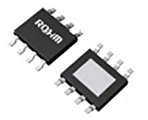HTSOP-J8 package: 4.90×6.00×1.00（mm）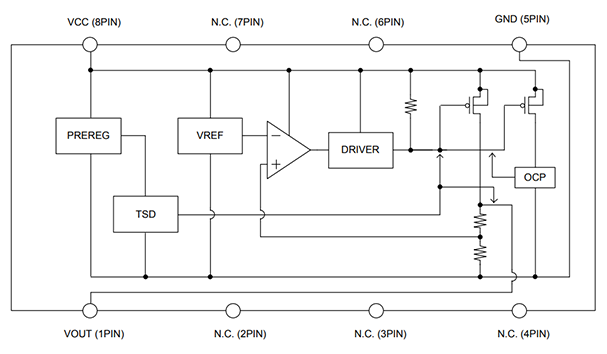### Calculation Example

The following condition settings are used to calculate the power consumption P.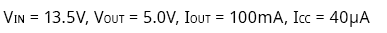※In the case of this IC, VCC is used for VIN.

The values are substituted into the equation for the power consumption P.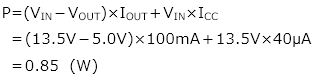Here TJ is calculated from the ambient temperature TA. θJA is determined from the graph, assuming that this IC is mounted on the PCB indicated below.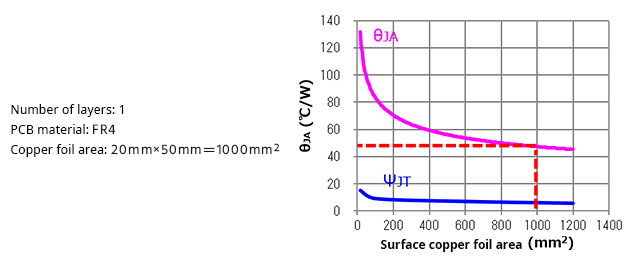From the graph, θJA is taken to be 48℃/W, a value for TA of 85℃ is assumed, and TJ is calculated for these conditions.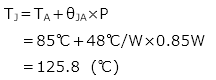As indicated in the specifications shown above, TJMAX for this IC is 150℃, so we can judge that these usage conditions are within the allowed range.

If the estimated value were higher than TJMAX, it would be necessary to modify the conditions. Modifications that could be made include reducing the power consumption P, lowering the ambient temperature TA, lowering the thermal resistance θJA, and the like. However, electrical specifications such as the input and output voltages and the output current are required conditions, and so changing them is generally difficult. It may in some cases be possible to lower TA by more intensive cooling or the like, but when TA is set as an operating specification for the equipment, changes are not easy. In order to lower θJA, in some cases it may be possible to expand the copper foil area of the PCB. When multiple types of IC packages are available, choosing a package with a lower θJA is another option. In any case, modifications to the PCB layout will also be required, so it is essential that TJ be carefully estimated in the design stage.

### Estimation of TJ through Actual Measurements

The above calculations are premised on the design conditions of the power supply; if the IC is already mounted on a PCB, TJ can also be estimated under close to actual conditions by measuring the power consumption P. As shown below, since IIN is equal to ICC+IOUT, VIN (VCC) ×IIN is the total input power to the IC, and this amount minus the output power consumption VOUT×IOUT is the power consumption P in the IC.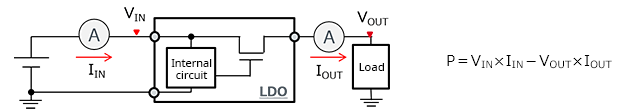The above is an example of calculations to estimate TJ using θJA. The method for calculating power consumption essentially appears on the IC data sheet, which should always be consulted.

In the next article, we present an example of calculation of TJ using ΨJT.